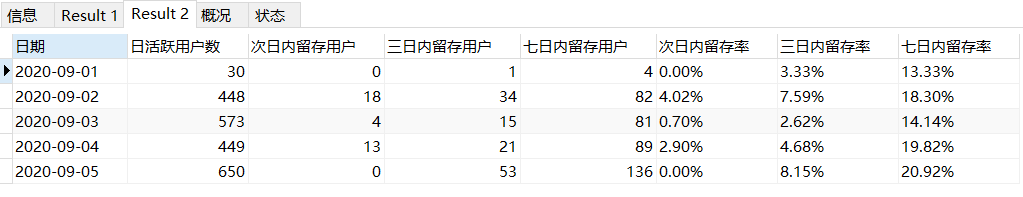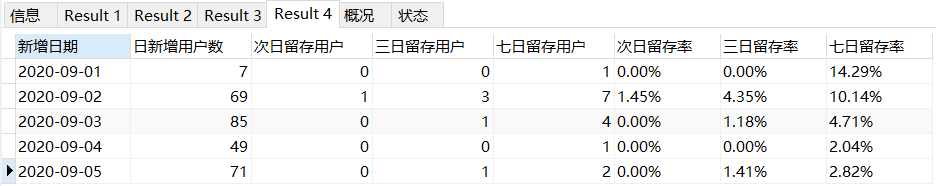# Mysql查询用户留存/留存率语法

• 计算某日的客户在第n日再次出现的概率--用户n日留存率。

• 计算某日的客户在某个时间段内再次出现的概率--用户n日内留存率。

• 计算某日新增的用户在第n日再次出现的概率--新用户n日留存率。

• 计算某日新增的用户在某个时间段内再次出现的概率--新用户n日内留存率。

## 1.用户n日留存/留存率

• 表名:订单总表;字段:客户编号,下单时间

``````-- 1.用户n日留存/留存率
-- 用户次日、3日、7日、30日,...留存/留存率

select *,
concat(round(100*次日留存用户/日活跃用户数,2),'%')  次日留存率,
concat(round(100*三日留存用户/日活跃用户数,2),'%')  三日留存率,
concat(round(100*七日留存用户/日活跃用户数,2),'%')  七日留存率
from (
select
date(a.下单时间) as 日期,
count(distinct a.客户编号) as 日活跃用户数,
count(distinct b.客户编号) as 次日留存用户,
count(distinct c.客户编号) as 三日留存用户,
count(distinct d.客户编号) as 七日留存用户
from 订单总表 a
left join 订单总表 b
on a.客户编号 = b.客户编号
and date(b.下单时间) = date(a.下单时间) + 1
left join 订单总表 c
on a.客户编号 = c.客户编号
and date(c.下单时间) = date(a.下单时间) + 3
left join 订单总表 d
on a.客户编号 = d.客户编号
and date(d.下单时间) = date(a.下单时间) + 7
where date(a.下单时间) between "2020/09/01" and "2020/09/05"
group by date(a.下单时间)
) p;
``````## 2.用户n日内留存/留存率

``````-- 2.用户n日内留存/留存率
-- 用户次日内、3日内、7日内、30日内,...留存/留存率

select *,
concat(round(100*次日内留存用户/日活跃用户数,2),'%')  次日内留存率,
concat(round(100*三日内留存用户/日活跃用户数,2),'%')  三日内留存率,
concat(round(100*七日内留存用户/日活跃用户数,2),'%')  七日内留存率
from (
select
date(a.下单时间) as 日期,
count(distinct a.客户编号) as 日活跃用户数,
count(distinct b.客户编号) as 次日内留存用户,
count(distinct c.客户编号) as 三日内留存用户,
count(distinct d.客户编号) as 七日内留存用户
from 订单总表 a
left join 订单总表 b
on a.客户编号 = b.客户编号
and date(b.下单时间) = date(a.下单时间) + 1
left join 订单总表 c
on a.客户编号 = c.客户编号
and (date(c.下单时间) BETWEEN date(a.下单时间) + 1 AND date(a.下单时间) + 3)
left join 订单总表 d
on a.客户编号 = d.客户编号
and (date(d.下单时间) BETWEEN date(a.下单时间) + 1 AND date(a.下单时间) + 7)
where date(a.下单时间) between "2020/09/01" and "2020/09/05"
group by date(a.下单时间)
) p;
``````## 3.新增用户n日留存/留存率

``````## 3.某日新增用户留存/留存率
## 计算某日新增的用户在次日、3日、7日的留存率--新用户n日留存

select *,
concat(round(100*次日留存用户/日新增用户数,2),'%')  次日留存率,
concat(round(100*三日留存用户/日新增用户数,2),'%')  三日留存率,
concat(round(100*七日留存用户/日新增用户数,2),'%')  七日留存率
from (
select
date(a.下单时间) as 新增日期,
count(distinct a.客户编号) as 日新增用户数,
count(distinct b.客户编号) as 次日留存用户,
count(distinct c.客户编号) as 三日留存用户,
count(distinct d.客户编号) as 七日留存用户
from
(
select
date(t1.下单时间) as 下单时间,
t1.客户编号
from 订单总表 as t1
left join 订单总表 as t2
on t1.客户编号=t2.客户编号
and t2.下单时间<t1.下单时间
where
(date(t1.下单时间) between "2020/09/01" and "2020/09/05")
and t2.下单时间 is Null
) as a
left join 订单总表 b
on a.客户编号 = b.客户编号
and date(b.下单时间) = date(a.下单时间) + 1
left join 订单总表 c
on a.客户编号 = c.客户编号
and date(c.下单时间) = date(a.下单时间) + 3
left join 订单总表 d
on a.客户编号 = d.客户编号
and date(d.下单时间) = date(a.下单时间) + 7
group by date(a.下单时间)
) p;
``````## 4.新增用户n日内留存率

``````## 4.新增用户n日内留存率
## 计算某日新增的用户在次日内、3日内、7日内的留存率--新用户n日内留存率。
select *,
concat(round(100*次日留存用户/日新增用户数,2),'%')  次日留存率,
concat(round(100*三日留存用户/日新增用户数,2),'%')  三日留存率,
concat(round(100*七日留存用户/日新增用户数,2),'%')  七日留存率
from (
select
date(a.下单时间) as 新增日期,
count(distinct a.客户编号) as 日新增用户数,
count(distinct b.客户编号) as 次日留存用户,
count(distinct c.客户编号) as 三日留存用户,
count(distinct d.客户编号) as 七日留存用户
from
(
select
date(t1.下单时间) as 下单时间,
t1.客户编号
from 订单总表 as t1
left join 订单总表 as t2
on t1.客户编号=t2.客户编号
and t2.下单时间<t1.下单时间
where
(date(t1.下单时间) between "2020/09/01" and "2020/09/05")
and t2.下单时间 is Null
) as a
left join 订单总表 b
on a.客户编号 = b.客户编号
and date(b.下单时间) = date(a.下单时间) + 1
left join 订单总表 c
on a.客户编号 = c.客户编号
and (date(c.下单时间) BETWEEN date(a.下单时间) + 1 AND date(a.下单时间) + 3)
left join 订单总表 d
on a.客户编号 = d.客户编号
and (date(d.下单时间) BETWEEN date(a.下单时间) + 1 AND date(a.下单时间) + 7)
group by date(a.下单时间)
) p;
``````### iOS_book 01- Xcode基本用法和Hello world_weixin_30613727的博客-程序员秘密

(基于精通iOS开发 第七版)iOS程序的特点1：在iOS中，一次只能有一个应用处于活动状态并显示在屏幕上。从iOS 4开始， 可以专门编写代码使用户按下home键后，app可以在后台继续运行。不处于活动状态或者在后台运行时，应用程序不会占用任何CPU资源，这会严重干扰其与开放式网络的链接。iOS允许后台处理，不过要使应用程序在此状况下良好运行则需要开发者自己努力。2：只能使...

daf

### Golang中 json.Decoder vs json.Unmarshal_json.unmarshal 数组_jason_cuijiahui的博客-程序员秘密

json的反序列化方式有两种：Use json.Unmarshal passing the entire response stringdata, err := ioutil.ReadAll(resp.Body)if err == nil &amp;amp;amp;amp;amp;amp;&amp;amp;amp;amp;amp;amp; data != nil { err = json.Unmarshal(data, value)}using json...

### 第一个react应用程序,初体验_#秃头小宝贝的博客-程序员秘密

react开发需要引入多个依赖文件，其中react.js、react-dom.js这两个文件是我们创建react应用程序必须要引入的依赖文件。react.js 是核心，提供创建元素，组件等功能https://unpkg.com/[email protected]/umd/react.development.jsreact-dom.js 提供DOM相关功能https://unpkg.com/[email protected]/umd/react-dom.development.js下载对应的react.js和re